Examples from NCERT Book

Class 10
Chapter 12 Class 10 - Electricity

## (c) the potential difference across the electric lamp and conductor.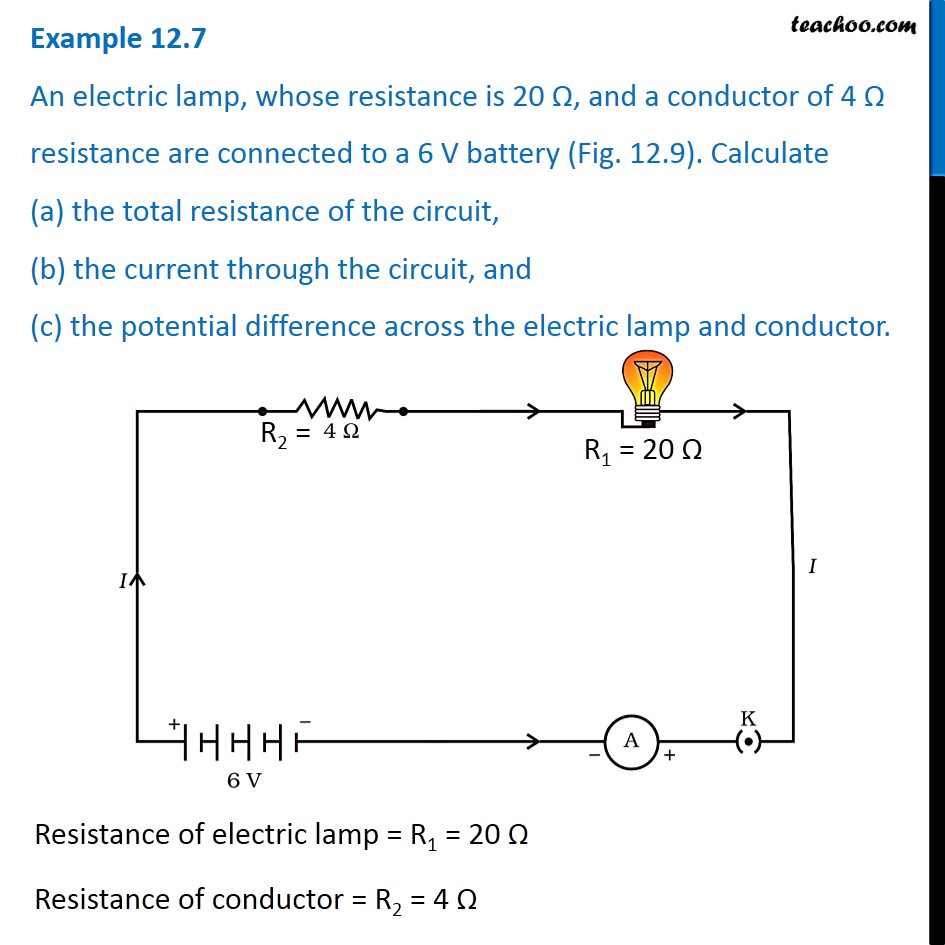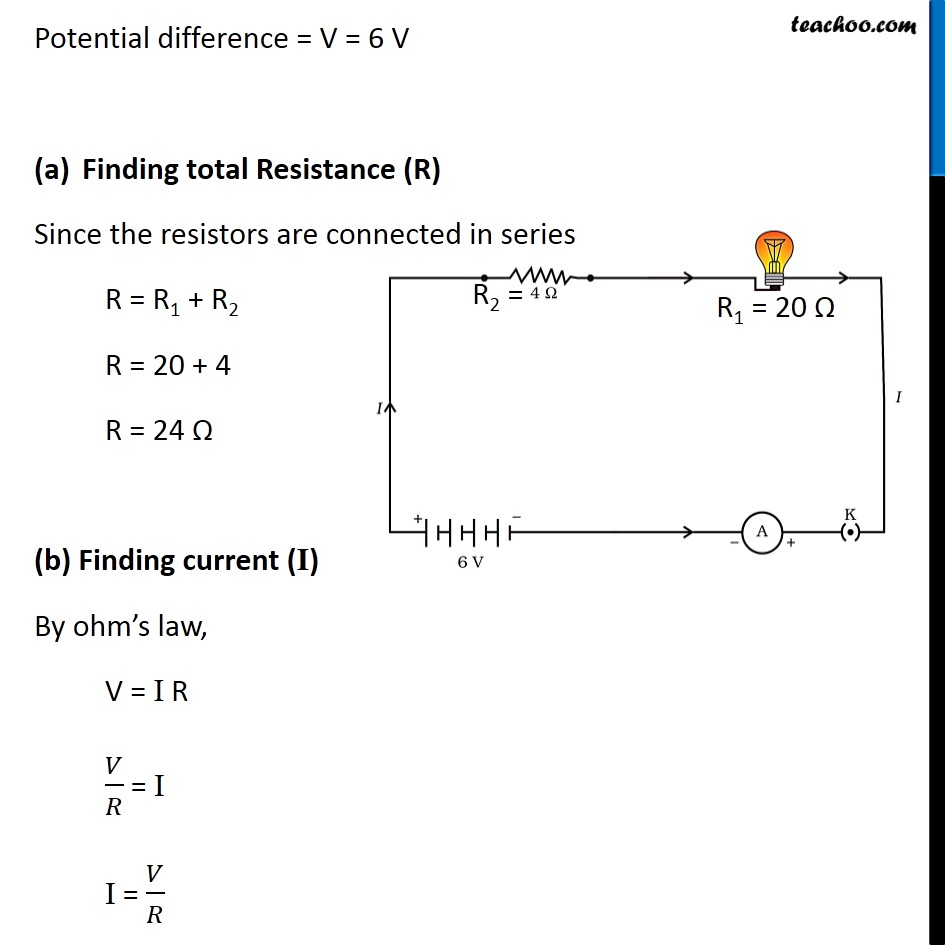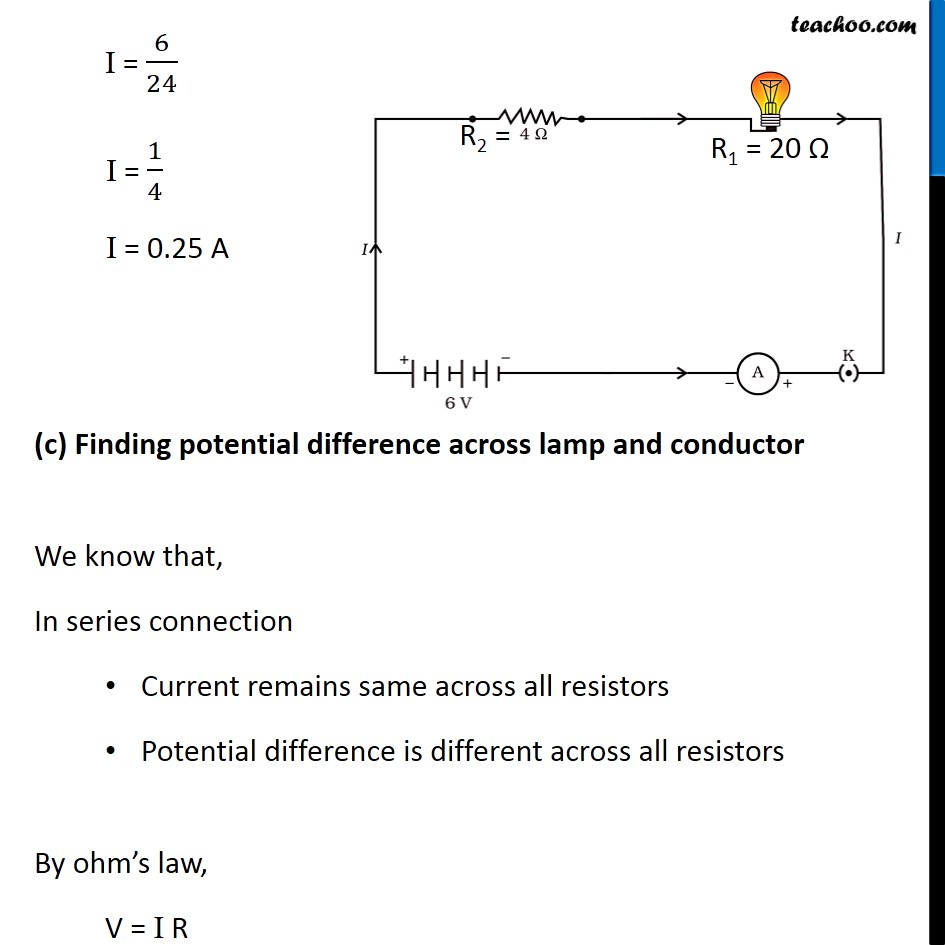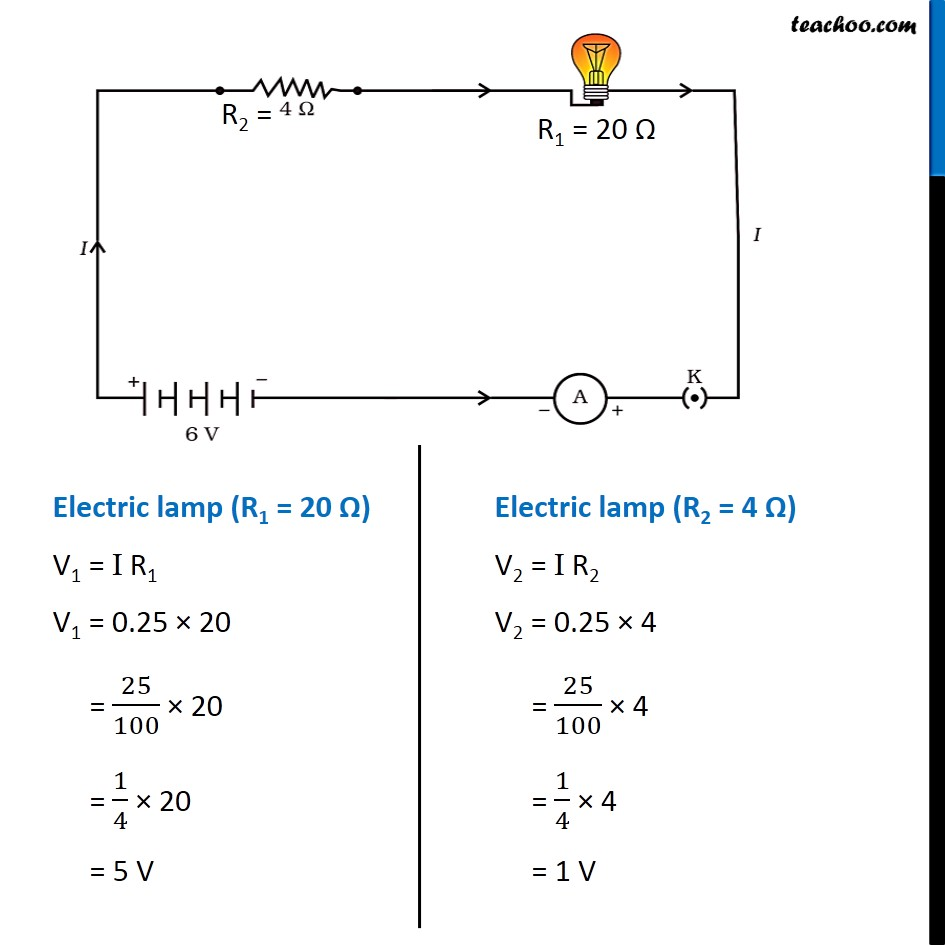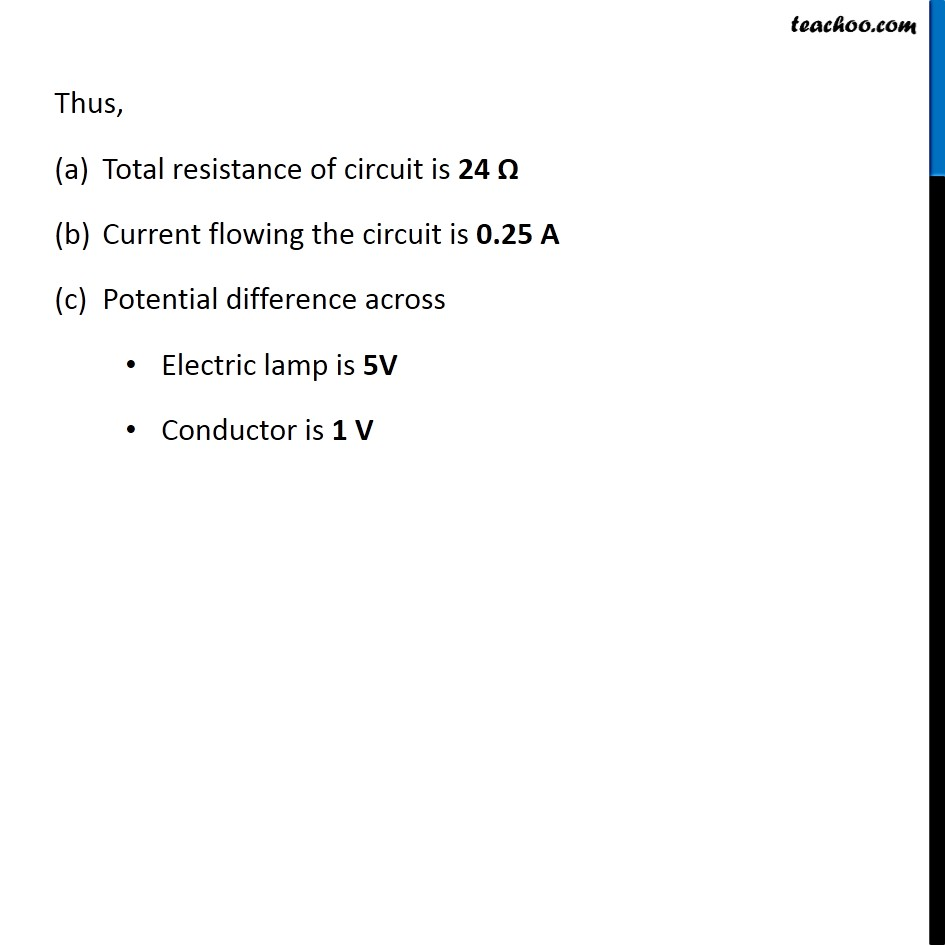Get live Maths 1-on-1 Classs - Class 6 to 12

### Transcript

Example 12.7 An electric lamp, whose resistance is 20 Ω, and a conductor of 4 Ω resistance are connected to a 6 V battery (Fig. 12.9). Calculate (a) the total resistance of the circuit, (b) the current through the circuit, and (c) the potential difference across the electric lamp and conductor. Resistance of electric lamp = R1 = 20 Ω Resistance of conductor = R2 = 4 Ω Potential difference = V = 6 V Finding total Resistance (R) Since the resistors are connected in series R = R1 + R2 R = 20 + 4 R = 24 Ω (b) Finding current (I) By ohm’s law, V = I R 𝑉/𝑅 = I I = 𝑉/𝑅 I = 6/24 I = 1/4 I = 0.25 A (c) Finding potential difference across lamp and conductor We know that, In series connection Current remains same across all resistors Potential difference is different across all resistors By ohm’s law, V = I R Electric lamp (R1 = 20 Ω) V1 = I R1 V1 = 0.25 × 20 = 25/100 × 20 = 1/4 × 20 = 5 V Electric lamp (R2 = 4 Ω) V2 = I R2 V2 = 0.25 × 4 = 25/100 × 4 = 1/4 × 4 = 1 V Thus, Total resistance of circuit is 24 Ω Current flowing the circuit is 0.25 A Potential difference across Electric lamp is 5V Conductor is 1 V